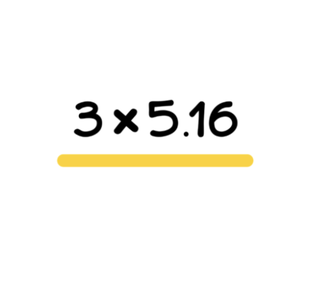Introduction to multiplication with decimals

# Introduction to multiplication with decimals

I will practice multiplying with decimal numbers.8,000 schools use Gynzy92,000 teachers use Gynzy1,600,000 students use Gynzy

## General

This lesson introduces the concept of multiplying decimal numbers and will start with whole numbers (one-digit) multiplied by decimal numbers (with tenths). Students will learn two different strategies to solve these problems. They will be encouraged to talk through their process and reflect on how it went.

## Standards

CCSS.MATH.CONTENT.5.NBT.B.7

## Learning objective

Students will be able to multiply with decimal numbers.

## Introduction

Students will be split into two teams and compete to see who can answer multiplication problems the fastest. Multiplying with decimal numbers is an important skill so students could, for example, figure out the distance they've biked.

## Instruction

The lesson will model how to multiply 3 × 2.7. It shows two different ways to arrive at the correct answer. Then, students will practice multiplying one-digit numbers whole numbers by decimal numbers with tenths (e.g., 2 × 4.2). They will complete two word problems and talk through their process while solving.

## Quiz

Students will complete 9 decimal multiplication questions.

## Closing

Students will be presented with decimal multiplication equations and will check the provided answers.

### The online teaching platform for interactive whiteboards and displays in schools

• Save time building lessons

• Manage the classroom more efficiently

• Increase student engagement